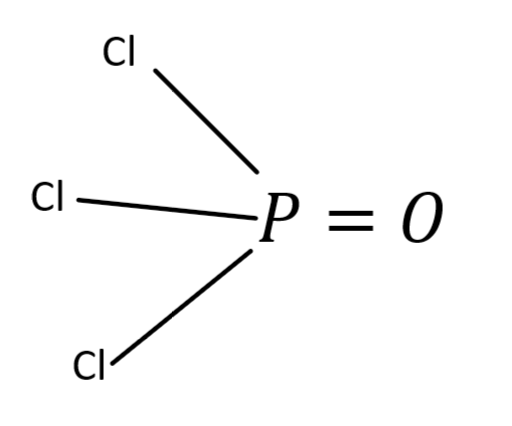Courses
Courses for Kids
Free study material
Free LIVE classes
MoreLIVE
Join Vedantu’s FREE Mastercalss

# The hybridization of $P$ in $POC{l_3}$ and shape of $POC{l_3}$ are, respectivelyA.$s{p^3}$, tetrahedralB.$s{p^3}$, pyramidalC.$s{p^3}$, square planarD.$s{p^3}$, distorted tetrahedralVerified
268.1k+ views
Hint: At first you should know about hybridization and the process to draw the structures of compounds. Hybridization is defined as the concept of mixing two atomic orbitals with the same energy levels to give new degenerate orbitals.

In order to determine the hybridization, we need to draw the structure of $POC{l_3}$. To form a bond between the two atoms there should be sharing of electron pairs between the atoms. As we all know that chlorine has one lone pair and oxygen has two lone pairs of atoms, the phosphorus atom forms three single bonds with three chlorine atoms and a double with one oxygen atom.From the above diagram, we can say that P has $3\sigma$ bonds with three chlorine atoms and it formed a double bond with an oxygen atom. We all know that a double bond contains one $\sigma$ and one $\pi$ bond.
Hybridization of an atom can also be calculated by using no of $\sigma$bonds that the atom is attached to. So from the above diagram the P atom is attached to $4{\sigma _{}}$ bonds then the hybridization of P atom is $s{p^3}$.
The shape of $POC{l_3}$is tetrahedral due to the asymmetric charge distribution around the phosphorus atom.